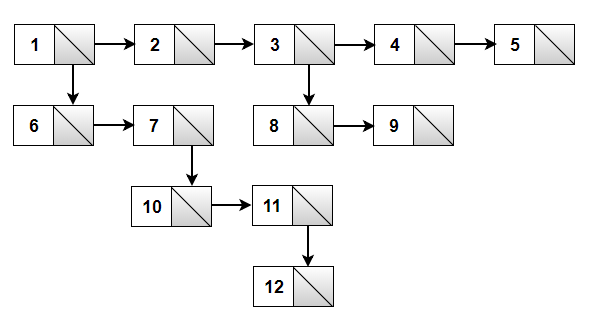# Convert a multilevel linked list to a singly linked list

Given a multilevel linked list, convert it into a singly linked list in such a way that all nodes of first level appears first, followed by all nodes of second level, and so on.

The multilevel linked list is similar to the simple linked list except that it has one extra field which points to the child of that node. The child may point to a separate list altogether, which may have children of its own.

For example, consider below multilevel linked listIt should be converted to list 1->2->3->4->5->6->7->8->9->10->11->12->null

The idea is to use queue data structure to solve this problem. We start by traversing the list horizontally from the head node using the next pointer, and whenever a node with a child is found, insert the child node in a queue. If end of the list is reached, we pop front node from the queue, set it as next node of last encountered node and repeat the entire process till queue becomes empty. This approach is demonstrated below in C++/Java –

## C++

Output:

1 -> 2 -> 3 -> 4 -> 5 -> 6 -> 7 -> 8 -> 9 -> 10 -> 11 -> 12 -> null

## Java

Output:

1 -> 2 -> 3 -> 4 -> 5 -> 6 -> 7 -> 8 -> 9 -> 10 -> 11 -> 12 -> null

The time complexity of above solution is O(n) where n is the number of nodes in the multilevel linked list.

Above solution requires O(n) extra space for queue data structure. We can also solve this problem with constant space. The idea is to maintain a tail pointer which always points to the end of the current list. Like previous approach, we start by traversing the list horizontally using next pointer. Now whenever we encounter a child node, we append it to the end of the list and update tail to last node of the child node. We repeat this process until we reach end of the list.

This is demonstrated below in C++/Java –

## C++

Output:

1 -> 2 -> 3 -> 4 -> 5 -> 6 -> 7 -> 8 -> 9 -> 10 -> 11 -> 12 -> nullptr

## Java

Output:

1 -> 2 -> 3 -> 4 -> 5 -> 6 -> 7 -> 8 -> 9 -> 10 -> 11 -> 12 -> null(1 votes, average: 5.00 out of 5)Loading...